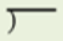# Access Point #: MAFS.4.NBT.2.AP.6a (Archived Access Point)

This document was generated on CPALMS - www.cpalms.org

Find whole-number quotients and remainders with up to three-digit dividends and one-digit divisors, using two different strategies.

#### Essential Understandings

Concrete:

• Given an expression, identify the dividend and the divisor (dividend = the total amount, divisor = number of groups or the number in each group).
• Use base ten blocks to model the dividend.
• Use base ten blocks to divide into groups determined by the divisor in order to find the quotient.
Representation:
• Symbols ÷, =,• Understand the following concepts and vocabulary of division, part, whole, divisor, dividend, quotient.
• Given a division expression, find the array using a jig on a pictorial representation to solve.

 Number: MAFS.4.NBT.2.AP.6a Category: Access Points Cluster: Use place value understanding and properties of operations to perform multi-digit arithmetic. (Major Cluster) :  Clusters should not be sorted from Major to Supporting and then taught in that order. To do so would strip the coherence of the mathematical ideas and miss the opportunity to enhance the major work of the grade with the supporting clusters.

#### Related Standards

Name Description
MAFS.4.NBT.2.6: Find whole-number quotients and remainders with up to four-digit dividends and one-digit divisors, using strategies based on place value, the properties of operations, and/or the relationship between multiplication and division. Illustrate and explain the calculation by using equations, rectangular arrays, and/or area models.
 Clarifications:Examples of Opportunities for In-Depth Focus When students work toward meeting this standard, they combine prior understanding of multiplication and division with deepening understanding of the base-ten system of units to find whole-number quotients and remainders with up to four-digit dividends and one- digit divisors. This work will develop further in grade 5 and culminate in fluency with the standard algorithms in grade 6.

#### Related Courses

 Name Description 5012060: Mathematics - Grade Four 7712050: Access Mathematics Grade 4 5012065: Grade 4 Accelerated Mathematics 5012015: Foundational Skills in Mathematics 3-5

#### Element Cards

 Name Description 4th Grade Math Element Cards: Element Cards are available to assist in planning for instruction. They are designed to promote understanding of how students move toward the academic standards. Element Cards contain one or more access points, essential understandings, suggested instructional strategies and suggested supports.